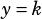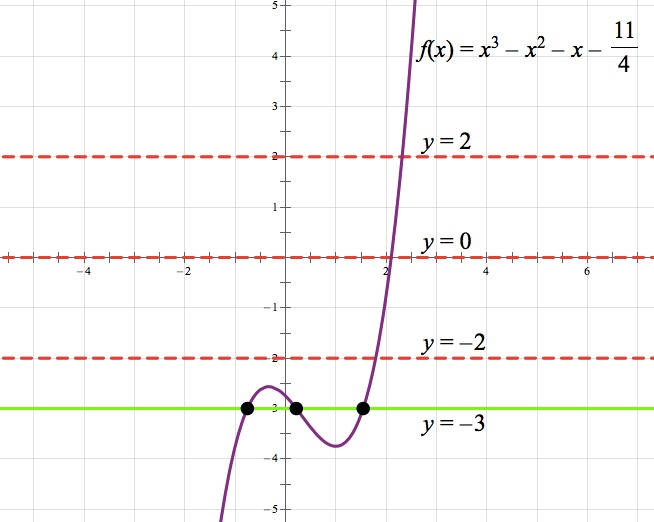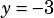Test #4 Section #4 Question #30!When they sayhas three real solutions, that means that theand thegraph intersect three times. Sincemust be a horizontal line because k is a constant, all we’re looking for here is where we can draw a horizontal line that will intersect the given graph three times. With that explanation, it might suddenly be obvious that the answer is –3, but in the image below I’ve graphed all answer choices.See how theline is the only one that intersects the graph three times? That’s why –3 is the answer.